# Direct relationship - 6th grade (11y) - math problems

#### Number of problems found: 101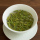If 4 and a half teaspoons of baking powder are need to make 10 servings of cornbread, how many teaspoons are needed to make 25 servings?
• Jewelry box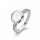The bottom of Zeyda's jewelry box is a rectangle with the length of 5 3/8 inches and a width of 3 1/4 inches. What is the area of the bottom of the jewelry box?
• A family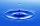A family of four consumes 220 liters of water a day. How many hectoliters of water are consumed by 68 tenants per day?
• Tennis balls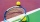Can of tennis balls contains 3 balls per can and cost \$7 how much will it cost for 36 tennis balls?
• The orchard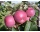4 temporary workers harvested the orchard in 9 days. How many temporary workers we need for six days?
• Milk2cheese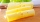From 40 liters of milk is produced 8 kg of cheese how many liters of milk are needed to produce 2 kg of cheese?
• A filterIt is a pool with a volume of 3500 liters. The filter filters at 4m cubic per hour. How many minutes would it filter the entire pool?
• The perimeter 3The perimeter of a rectangle is 35 cm. The ratio of the length to its width is 3:2. Calculate the dimensions of the rectangle
• Cyclist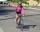Cyclist drives 5 km in half an hour, how long will drive in 3 hours?
• Pump680 liters of water were pumped in 8 minutes. How many liters was spent in 56 minutes?
• Land area 2A land area was divided among the three heirs in the ratio 5:2:4. If the largest share was 20 hectares of land, what is the total area of land? Please show your solution and what kind of proportion is this please
• Reducing scale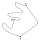I am sizing some landscaping and I measured everything for a 20 to 1 scale. I realized when I was done that it was at 30 to 1. How can I convert what I already have to 30 to 1. (Example, grass area is 22,871.6' at 20 to 1, how do you figure it out to be 3
• Michal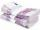Michal has 2.90 per hour. How much does he earn during the week of the summer job?
• Two brothersThe two brothers were to be divided according to the will of land at an area of 1ha 86a 30m2 in a ratio of 5:4. How many will everyone get?
• On the mapA line 1.5 cm long corresponds to a line 3 cm long on the map. What is the scale of the map?
• BuildingAt the building, we divided 240 boards into two piles in a 5: 3 ratio. How many were fewer boards in the lower pile?
• Report 2A School reports students to teacher ratio of 6:1. If there are 45 teachers in the School, how many students are there?
• Map scaleThe rectangular plot has a scale of 1: 10000 area 3 cm2 on the map. What content does this plot have on a 1:5000 scale map?
• Cylinder-shaped vase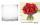If the cylinder-shaped vase is filled with water up to 35 cm, it contains 1 liter of water. How much water will it contain if it is filled to a height of 45cm?
• Babysitting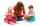The amount that Susan charges per hour for babysitting are directly proportional to the number of children she is watching. She charges \$4.50 for 3 kids. How much would she charge to babysit 5 kids?

Do you have an exciting math question or word problem that you can't solve? Ask a question or post a math problem, and we can try to solve it.

We will send a solution to your e-mail address. Solved examples are also published here. Please enter the e-mail correctly and check whether you don't have a full mailbox.

Direct relationship - math problems. Examples for 6th grade (the sixth graders).